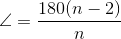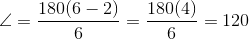# High School Math : How to find an angle in a hexagon

## Example Questions

210

170

180

190

200

### Example Question #2 : How To Find An Angle In A Hexagon

If a triangle has 180 degrees, what is the sum of the interior angles of a regular octagon?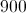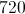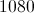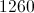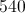Explanation:

The sum of the interior angles of a polygon is given by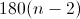where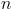= number of sides of the polygon.  An octagon has 8 sides, so the formula becomes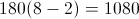### Example Question #1 : How To Find An Angle In A Hexagon

What is the measure of an interior angle of a regular hexagon?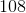Explanation:

The measure of an internal angle of an regular polygon can be determined using the following equation, whereequals the number of sides: